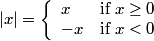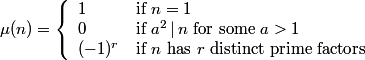# How to write multi-part definitions in LaTeX

This post explains how to typeset multi-part definitions in LaTeX.

The absolute value function is a simple example of a two-part definition.The Möbius function is a more complicated example of a three-part definition.Here’s how you could write LaTeX for the absolute value definition.

|x| =
\left\{
\begin{array}{ll}
x  & \mbox{if } x \geq 0 \\
-x & \mbox{if } x < 0
\end{array}
\right.

The right-hand side of the equation is an array with an opening brace sized to fit on the left. Braces are special characters and so the opening brace needs to be escaped with a backslash. LaTeX requires a right for every left but the dot in right. says to make the matching container on the right side empty.

Since this pattern comes up fairly often, it’s handy to have a command to encapsulate it. We define twopartdef as follows.

\newcommand{\twopartdef}
{
\left\{
\begin{array}{ll}
#1 & \mbox{if } #2 \\
#3 & \mbox{if } #4
\end{array}
\right.
}

Then we could call it as follows:

|x| = \twopartdef { x } {x \geq 0} {-x} {x < 0}

The command threepartdef is very similar to twopartdef.

\newcommand{\threepartdef}
{
\left\{
\begin{array}{lll}
#1 & \mbox{if } #2 \\
#3 & \mbox{if } #4 \\
#5 & \mbox{if } #6
\end{array}
\right.
}

You could call threepartdef for the Möbius function as follows.

mu(n) = \threepartdef
{1}      {n=1}
{0}      {a^2 ,|, n \mbox{ for some } a > 1}
{(-1)^r} {n \mbox{ has } r \mbox{ distinct prime factors}}

## 19 thoughts on “How to write multi-part definitions in LaTeX”

1. John,
This functionality is provided natively by LaTeX in the “cases” environment. It’s described in the LaTeX Companion (sec. 8.4.1).

Example:

|x| =
begin{cases}
x & text{if } x geq 0 \
-x & text{if } x < 0
end{cases}

(Editors note: To use the “cases” environment, add usepackage{amsmath} to the preamble. — JC)

2. Thanks. I didn’t know that.

The “cases” environment wasn’t part of LaTeX when I learned it, back before version 2e. Back in my day … :)

3. Daniel Lemire

I also hacked something similar for a recent paper of mine. I also did not know about the cases environment, though I’m a long time LaTeX user.

4. Alasdair

If you’re using LaTeX for maths (which you are!) then you should automatically also use the amsmath package, which contains many many commands for different sorts of displayed equations, of which cases is one. Not all of amsmath is part of standard LaTeX.

5. The cases environment will be a bit higher than the array environment, you could see if you would compare the examples above, because there’s a fixed arraystretch of 1.2 internally. It may be ok, but if you want to stretch it less or more according to the content here’s a possibility, amsmath: cases and arraystretch.

6. Lilian

Hello, it is nice the amsmath contains several math functions, but for multi-part fuctions, I actually prefer John’s solution. You have to type less than using “case”.

7. Lilian, you can use John’s solution (i. e. defining a custom command) with cases as well ;-)

8. Ibrahim

How do I generate a numbered “Definition” environment that has multiple options in LaTeX? For example:

Definition 1.2 (Mathematics)
: is the study of patterns and structures.
: is the manipulation of numbers.

10. Derik Evangelista

Thank you! Very usefull.

11. Patrick Goetz

One tiny bug fix to the original solution. The use of mbox in this context doesn’t make sense — use text{} instead:

|x| =
left{
begin{array}{ll}
x & text{if } x geq 0 \
-x & text{if } x < 0
end{array}
right.

12. Patrick Goetz

Addendum: just realized that text is part of the amsmath package, so you need to have this loaded for text to work. However, as far as I can tell, everyone should be using amsmath, at this point anyway (but at least now I understand why the original recipe used mbox instead).

13. Alex Chan

You can also use the dcases package (loaded with mathtools) if you want the vertical spacing of your environment to stretch accordingly (for example, if you had displayed fractions on one line).

The mathtools package has quite a few environments in a similar vein for handling cases well, such as rcases (the brace goes on the right) and dcases* (where the right hand text is typeset in the document’s roman font, not as maths).

Package documentation: http://mirror.math.ku.edu/tex-archive/macros/latex/contrib/mh/mathtools.pdf

14. Abdoulaye

I think there is a typo in the code using array, we should put ‘\left\{‘ instead of ‘\left{‘ which was causing error in my code…

15. Thanks. I recently upgraded my blog and lost some backslash characters in the process. I added back in the backslashes manually and I missed a few.

16. Hi,
I was searching how an equation could be written into multiple lines without using amsmath package. Your solution really works! I learned much more than writing a multi-line equation from you.
Thank you John.

17. Thanks. Glad it was useful.

18. Benjamin

This has already been helpful in typesetting some equations for my Ph.D. thesis. Thank you!

19. This is rather called “piecewise defined” function/expression. When I read “multi-part definition” I thought of nested/modular/interdependent \def’s…